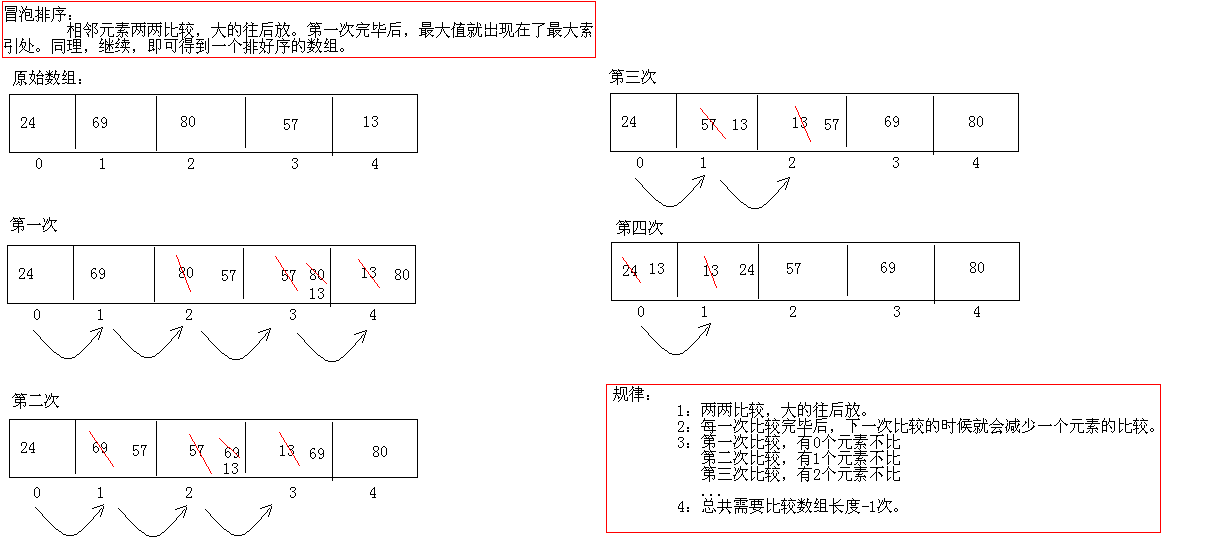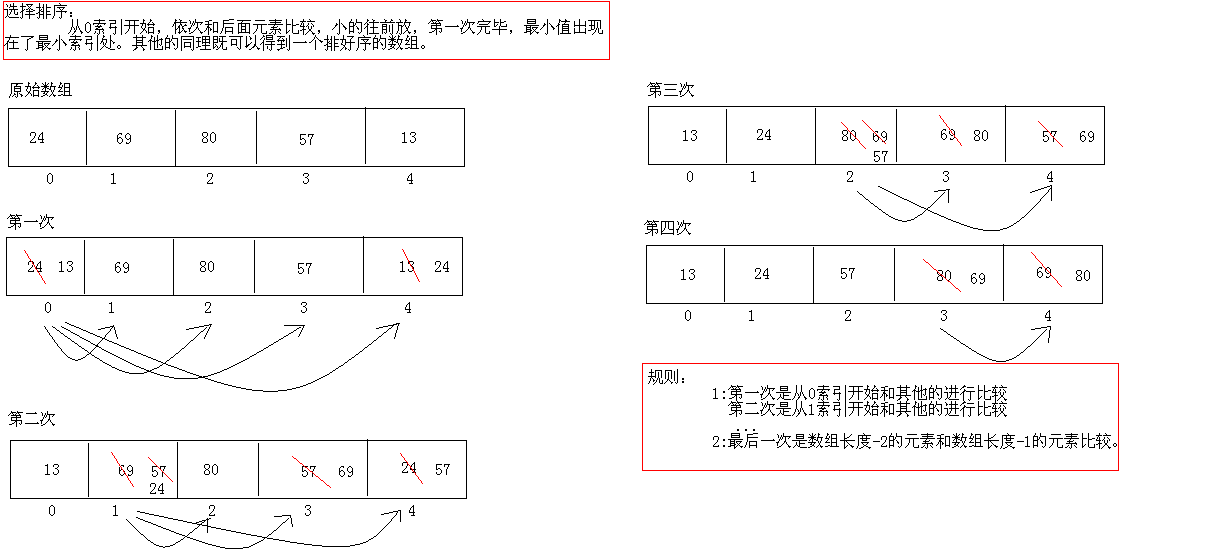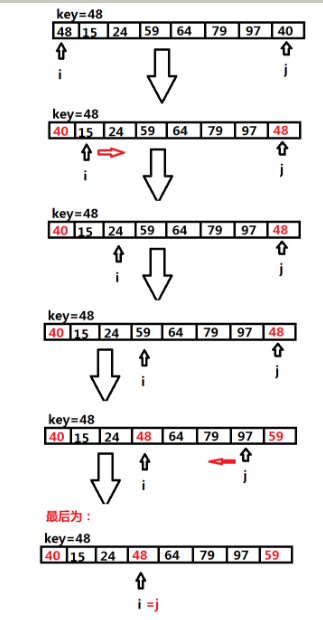# 各种排序总结（一）之--—冒泡排序、选择排序、快速排序

各种排序总结——冒泡排序、选择排序、快速排序

1、冒泡排序是比较经典的排序方法，是一种用时间换空间的排序方法。我总结了一下它的特点：（1）它的时间复杂度是；（2）每一趟相邻元素两两比较完毕就会产生最值（最大值）；（3）每次比较完后下一趟就会少一个元素参与比较（即该趟比较的最大值）。package lianxi_01;

public class Bubble{
public static void main(String[] args){
int score[] = { 24, 69, 80, 57, 13 };
for (int i = 0; i < score.length -1; i++){    //最多做n-1趟排序
for(int j = 0 ;j < score.length - i - 1; j++){    //对当前无序区间score[0......length-i-1]进行排序(j的范围很关键，这个范围是在逐步缩小的)
if(score[j] < score[j + 1]){    //把小的值交换到后面
int temp = score[j];
score[j] = score[j + 1];
score[j + 1] = temp;
}
}
System.out.print("第" + (i + 1) + "次排序结果：");
for(int a = 0; a < score.length; a++){
System.out.print(score[a] + "\t");
}
System.out.println("");
}
System.out.print("最终排序结果：");
for(int a = 0; a < score.length; a++){
System.out.print(score[a] + "\t");
}
}
}  

2、选择排序相比冒泡排序不稳定，时间复杂度也是。选择排序没趟都会产生最小值，它不是相邻元素的比较而是在该元素设置一个索引i。然后与数组的其他元素依次比较（除了上一个索引值），直到找到小于该元素（索引j）时交换两元素，接着继续从i索引（此时已经不是原来的数值）值与索引j+1值比较。重复上述比较过程……简单的原理图如下：package lianxi_01;

/*
* 选择排序
*/
public class XuanzeDemo {
public static void main(String[] args) {
int score[] = { 24, 69, 80, 57, 13 };

for (int x = 0; x < score.length - 1; x++) {
for (int y = x + 1; y < score.length; y++) {
if (score[y] < score[x]) {
int temp = score[x];
score[x] = score[y];
score[y] = temp;
}
}
}

System.out.print("最终排序结果：");
for(int a = 0; a < score.length; a++){
System.out.print(score[a] + "\t");
}
}
}


3、快速排序

一趟快速排序的算法是：

1）设置两个变量i、j，排序开始的时候：i=0，j=N-1；

2）以第一个数组元素作为关键数据，赋值给key，即 key=A；

3）从j开始向前搜索，即由后开始向前搜索（j=j-1即j--）， 找到第一个小于key的值A[j]，A[i]与A[j]交换；

4）从i开始向后搜索，即由前开始向后搜索（i=i+1即i++）， 找到第一个大于key的A[i]，A[i]与A[j]交换；

5）重复第3、4、5步，直到I=J； (3,4步是在程序中没找到时候j=j-1，i=i+1，直至找到为止。 找到并交换的时候i， j指针位置不变。另外当i=j这过程一定正好是i+或j-完成的最后令循环结束）

这里的过程原理图比较复杂没有画出，下面是一个循环体的分析图：package lianxi_01;

import java.util.Arrays;

/*
* 快速排序
*/
public class QuickSortDemo {
public static void main(String[] args) {
//定义数组
int data[] = { 24, 69, 80, 57, 13 };

quickSort(data, 0, data.length - 1);

System.out.println("排序后：" +Arrays.toString(data));
}
private static void quickSort(int[] data, int i, int j) {
int pivotIndex = (i + j) / 2;
// swap
swap(data, pivotIndex, j);

int k = partition(data, i - 1, j, data[j]);
swap(data, k, j);
if ((k - i) > 1)
quickSort(data, i, k - 1);
if ((j - k) > 1)
quickSort(data, k + 1, j);

}
private static int partition(int[] data, int l, int r, int pivot) {
do {
while (data[++l] < pivot);
while ((r != 0) && data[--r] > pivot);
swap(data, l, r);
} while (l < r);
swap(data, l, r);
return l;
}

public static void swap(int[] data, int i, int j) {
int temp = data[i];
data[i] = data[j];
data[j] = temp;
}
}

（下一篇介绍插入排序、希尔排序、归并排序）。希望大家多多提出你们宝贵的建议哈©️2019 CSDN 皮肤主题: 大白 设计师: CSDN官方博客